Analysis WebNotes
arrow_back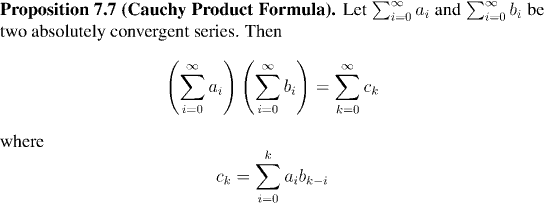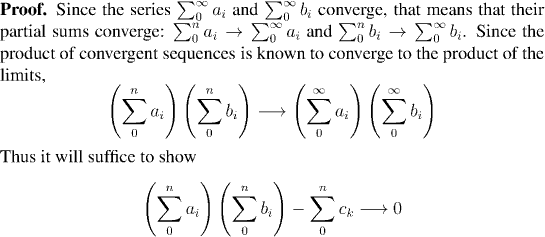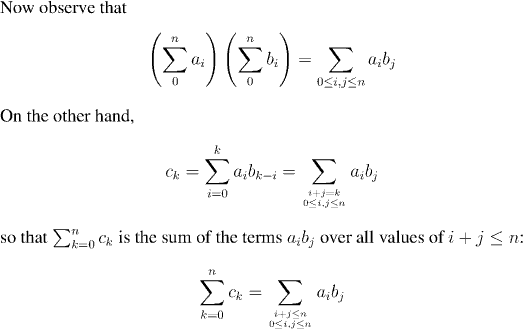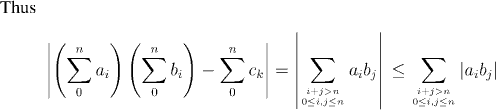We can think of the set of all i,j between 0 and n as the shaded region on the grid: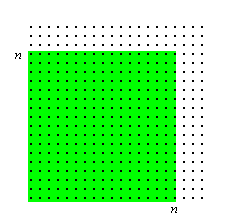Then the set of pairs that we sum over to get the sum of the ck's is: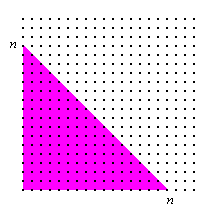The remaining terms are the summation over the region: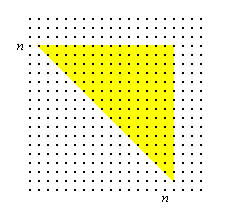We are trying to show that as n grows, the summation over this region goes to zero. The trick is to notice that although the region grows in size as n grows, the terms that belong to the region lie further and further out. We shall show that the upper triangular region lies inside the larger "L-shaped" region shown below, and make an estimate of the summation over that region.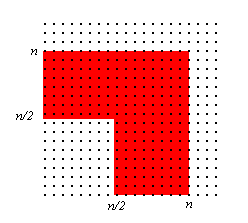(Notice for the estimate below that this last shape is obtained by taking a square of side n and deleting a square of side n/2 from the corner.)